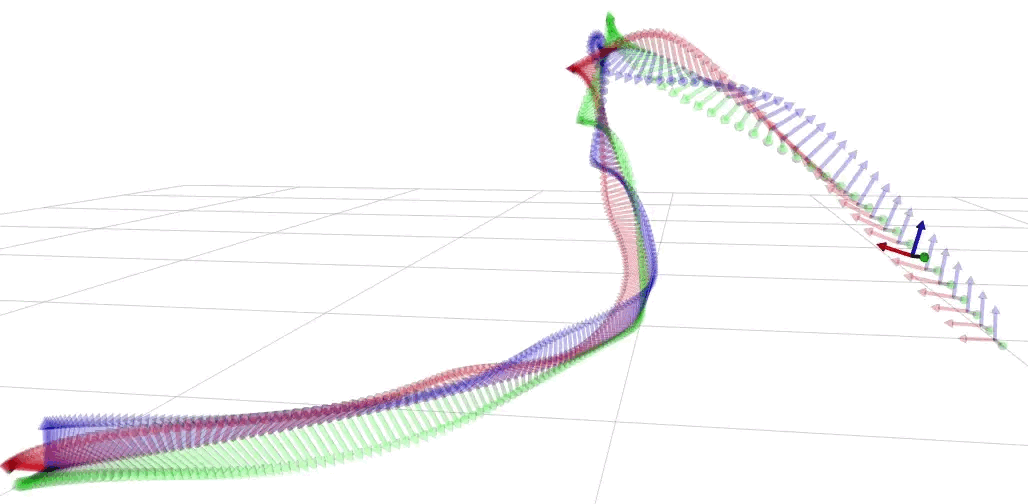# Geometric Algebra for Optimal Control with Application in Manipulation Tasks

## Tobias Löw and Sylvain Calinon

Idiap Research Institute/EPFL

Abstract Many problems in robotics are fundamentally problems of geometry, which lead to an increased research effort in geometric methods for robotics in recent years. The results were algorithms using the various frameworks of screw theory, Lie algebra and dual quaternions. A unification and generalization of these popular formalisms can be found in geometric algebra. The aim of this paper is to showcase the capabilities of geometric algebra when applied to robot manipulation tasks. In particular the modelling of cost functions for optimal control can be done uniformly across different geometric primitives leading to a low symbolic complexity of the resulting expressions and a geometric intuitiveness. We demonstrate the usefulness, simplicity and computational efficiency of geometric algebra in several experiments using a Franka Emika robot. The presented algorithms were implemented in c++20 and resulted in the publicly available library gafro. The benchmark shows faster computation of the kinematics than state-of-the-art robotics libraries.

A general multivector in geometric algebra:The structure of a multivector in conformal geometric algebra:The outer product of two points results in a point pair.The outer product of two points and the point at infinity results in a line.Using the sandwich product of motors to transform a point results in another point. The operation is grade preserving.## ExperimentsAn oriented pointmass system.System tracking a point target.Pointing task, i.e. system tracking a point target using a line.System tracking a line target. It can be seen that the system is compliant if moved along the line and stiff if moved away from the line.